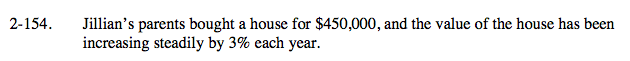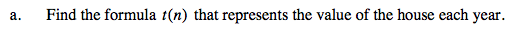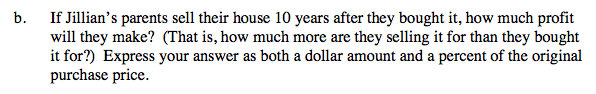Home > A2C > Chapter 2 > Lesson 2.2.3 > Problem2-154

2-154.
1. Jillian's parents bought a house for $450,000, and the value of the house has been increasing steadily by 3% each year. Homework Help ✎ 1. Find the formula t(n) that represents the value of the house each year. 2. If Jillian's parents sell their house 10 years after they bought it, how much profit will they make? (That is, how much more are they selling it for than they bought it for?) Express your answer as both a dollar amount and a percent of the original purchase price.Review the online help for problem 2-13. $V=450,000 \cdot (1.03)^\textit{t}$ Where V = value of the house ($)
t = time (years)After you find how much the house is worth after 10 years, do not forget to subtract the original price to find the profit.